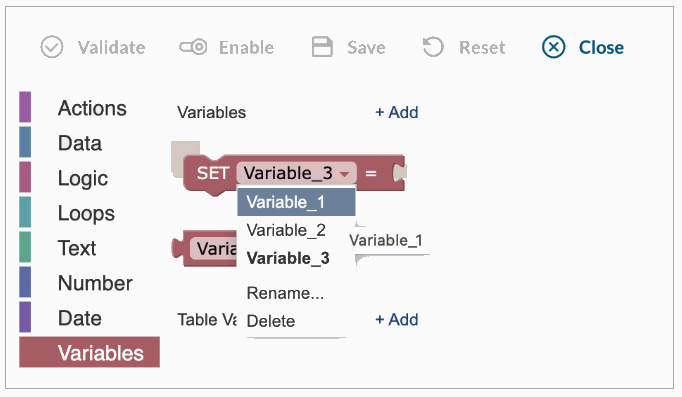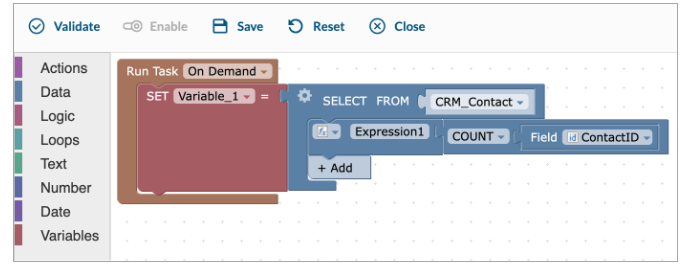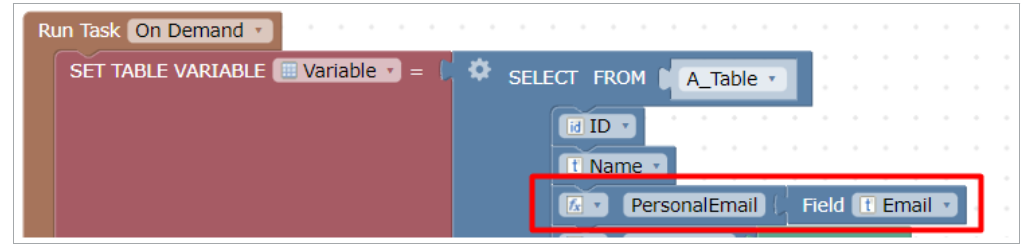Variables store information to be referenced in the trigger logic. There are two types of variables:

• variables for holding values or results of calculations,
• table variables for storing the set of records, which can be then used as the data source for other action blocks.

Steps to create a variable

1. In the Variables section, select Add and enter a name.2. Define the value for the variable by selecting the appropriate variable from the drop-down list.3. Drag the SET block and drop it into your trigger logic.4. Assign a value to the variable. Type the value or drag the appropriate function block from the left menu bar.
• A static value, the value of another variable or a calculation can be set for the variable.
• The variable value can be updated later in the calculations.
• The variable data type is identified automatically based on the value assigned. The following variable data types are supported: text, numeric, date, binary (yes/no).

Example: Variable_1 gets number of records from CRM_Contact table.1. Drag the variable value block and drop it into your trigger logic where you need to use the value of the variable.Note: Variables need to be defined first before being used in the trigger logic.

For table variables,

• The initial selection defines the table variable structure (the list of fields and their data type).
• It’s not allowed to use (SET) a table variable more than once even if SELECT is exactly the same for the second time.
• Since the table variable behaves like a table, the changes of its values (records and fields) are performed using regular UPDATE, INSERT, and DELETE blocks.
• You can change the column name of the table variable, for instance, to use this table variable in the email message. To do that, use an expression instead of the field itself for the column header.Note: Initializing a variable by assigning a value determines its data type and cannot be changed later in the logic.